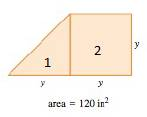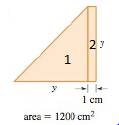# The value of y .### Precalculus: Mathematics for Calcu...

6th Edition
Stewart + 5 others
Publisher: Cengage Learning
ISBN: 9780840068071### Precalculus: Mathematics for Calcu...

6th Edition
Stewart + 5 others
Publisher: Cengage Learning
ISBN: 9780840068071

#### Solutions

Chapter 1.6, Problem 46E

(a)

To determine

## To find: The value of y.

Expert Solution

The value of y is 45in .

### Explanation of Solution

Given:

The given figure is,Figure (1)

The area of figure is 120in2 .

Calculation:

Tabulate the given information into the language of algebra.

 In words In Algebra Base of part 1 y Height of part 1 y Area of part 1 y×y2 Length of part 2 y Area of part 2 y×y

Model the equation for the above information.

Areaofpart1+Areaofpart2=Totalareay×y2+y×y=120

Simplify the above equation for y.

y22+y2=120y2+2y2=2403y2=240y=45

Thus, the value of y is 45in .

(b)

To determine

### To find: The value of y.

Expert Solution

The value of y is 5 in.

### Explanation of Solution

Given:

The given figure is,Figure (2)

The area of figure is 1200cm2 .

Calculation:

Tabulate the given information into the language of algebra.

 In words In Algebra Width of part 1 y Length of part 1 y Area of part 1 y×y Length of part 2 y Area of part 2 y×1

Model the equation for the above information.

Areaofpart1+Areaofpart2=Totalareay×y2+y×1=1200

Simplify the above equation for y.

y22+y=1200y2+2y=2400y2+2y2400=0

Further simplify the above equation.

(y+50)(y48)=0y=50,y=48

The value of x cannot be negative.

Thus, the value of y is 48 cm.

### Have a homework question?

Subscribe to bartleby learn! Ask subject matter experts 30 homework questions each month. Plus, you’ll have access to millions of step-by-step textbook answers!